# Interpret the key results for Correlation

Complete the following steps to interpret a correlation analysis. Key output includes the Pearson correlation coefficient, the Spearman correlation coefficient, and the p-value.

## Step 1: Examine the relationships between variables on a matrix plot

Use the matrix plot to examine the relationships between two continuous variables. Also, look for outliers in the relationships. Outliers can heavily influence the results for the Pearson correlation coefficient.

Determine whether the relationships are linear, monotonic, or neither. The following are examples of the types of forms that the correlation coefficients describe. The Pearson correlation coefficient is appropriate for linear forms. Spearman's correlation coefficient is appropriate for monotonic forms.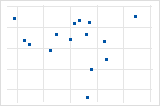No relationshipThe points fall randomly on the plot, which indicates that there is no linear relationship between the variables.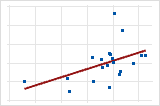Moderate positive relationshipSome points are close to the line but other points are far from it, which indicates only a moderate linear relationship between the variables.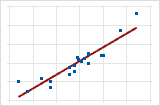Large positive relationshipThe points fall close to the line, which indicates that there is a strong linear relationship between the variables. The relationship is positive because as one variable increases, the other variable also increases.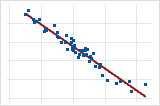Large negative relationshipThe points fall close to the line, which indicates that there is a strong negative relationship between the variables. The relationship is negative because, as one variable increases, the other variable decreases.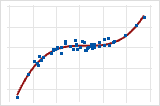Monotonic In a monotonic relationship, the variables tend to move in the same relative direction, but not necessarily at a constant rate. In a linear relationship, the variables move in the same direction at a constant rate. This plot shows both variables increasing concurrently, but not at the same rate. This relationship is monotonic, but not linear. The Pearson correlation coefficient for these data is 0.843, but the Spearman correlation is higher, 0.948.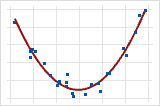Curved quadraticThis example shows a curved relationship. Even though the relationship between the variables is strong, the correlation coefficient would be close to zero. The relationship is neither linear nor monotonic.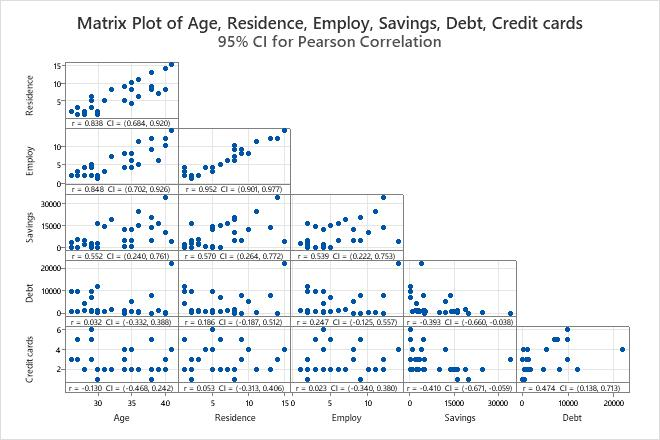Key Result: Matrix PlotIn these results, you can see positive linear relationships, negative linear relationships, possible curved relationships, and a few outliers.A strong positive linear relationship exists between Employ and Residence. A weak negative linear relationship exists between Credit cards and Savings. Debt seems to have an outlier which should be investigated.

## Step 2: Examine the correlation coefficients between variables

Use the Pearson correlation coefficient to examine the strength and direction of the linear relationship between two continuous variables.

Strength

The correlation coefficient can range in value from −1 to +1. The larger the absolute value of the coefficient, the stronger the relationship between the variables.

For the Pearson correlation, an absolute value of 1 indicates a perfect linear relationship. A correlation close to 0 indicates no linear relationship between the variables.
Direction

The sign of the coefficient indicates the direction of the relationship. If both variables tend to increase or decrease together, the coefficient is positive, and the line that represents the correlation slopes upward. If one variable tends to increase as the other decreases, the coefficient is negative, and the line that represents the correlation slopes downward.

Consider the following points when you interpret the correlation coefficient:
• It is never appropriate to conclude that changes in one variable cause changes in another based on correlation alone. Only properly controlled experiments enable you to determine whether a relationship is causal.
• The Pearson correlation coefficient is very sensitive to extreme data values. A single value that is very different from the other values in a data set can greatly change the value of the coefficient. You should try to identify the cause of any extreme value. Correct any data entry or measurement errors. Consider removing data values that are associated with abnormal, one-time events (special causes). Then, repeat the analysis.
• A low Pearson correlation coefficient does not mean that no relationship exists between the variables. The variables may have a nonlinear relationship.
By using this site you agree to the use of cookies for analytics and personalized content.  Read our policy